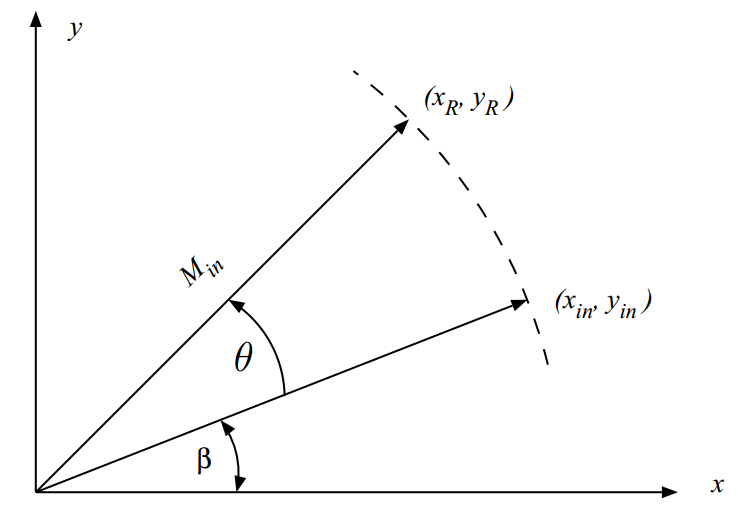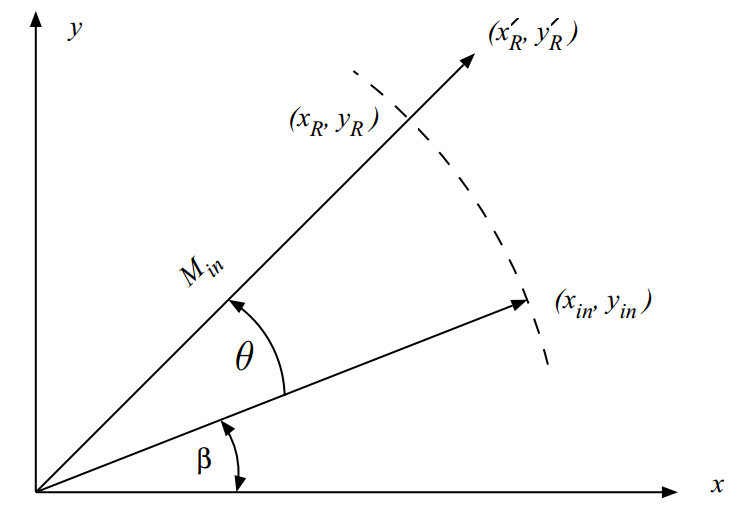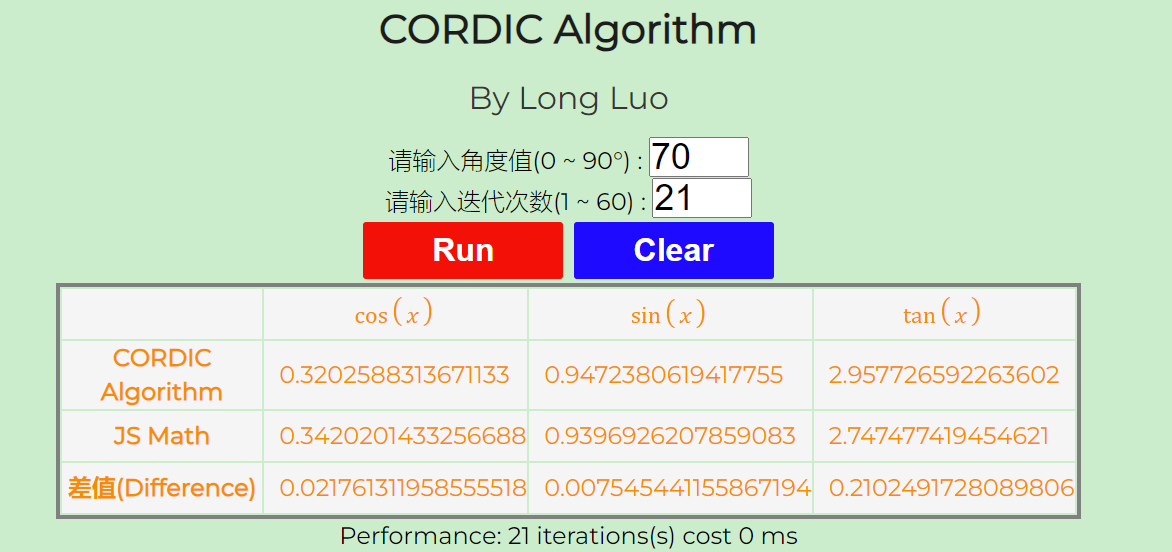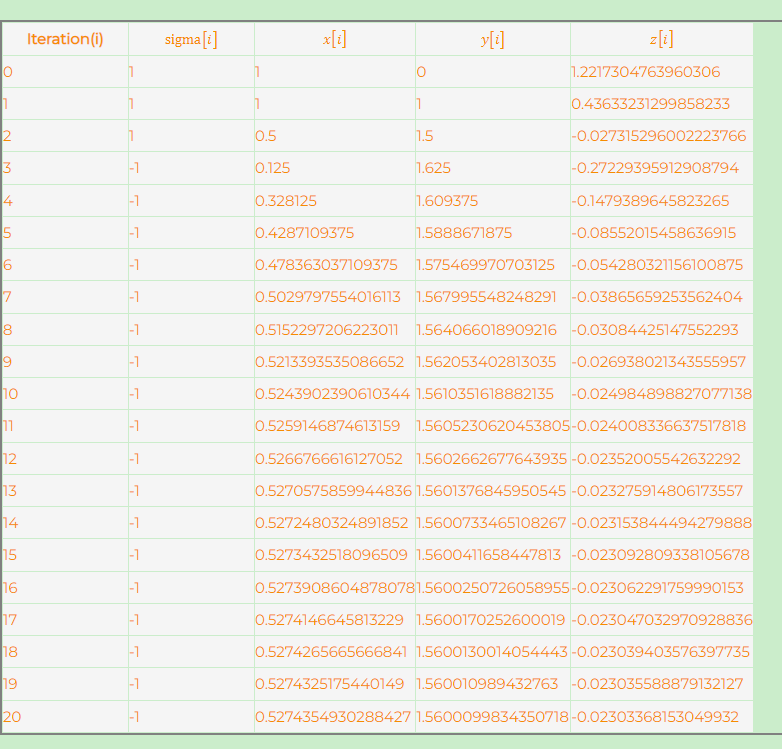By Long Luo

$\sin \angle 18^{\circ} = \frac{\sqrt {5} - 1}{4} \approx 0.3090169943$

$\sin x \approx x - \frac{x^3}{3!} + \frac{x^5}{5!} - \frac{x^7}{7!}$

$\sin \angle 18^{\circ} = \sin \frac{\pi}{10} = \frac{\pi}{10} - \frac{1}{6} (\frac{\pi}{10})^3 + \frac{1}{120} (\frac{\pi}{10})^5 - \frac{1}{5040} (\frac{\pi}{10})^7 \approx 0.30903399538$

# 泰勒公式的缺点

1. 展开过小则会导致展开精度不够，展开过大则会导致计算量过大；
2. 幂运算需要使用乘法器，存在很多重复计算；
3. 需要很多变量来存储中间值。

# 解析 CORDIC 算法$x_R = x_{in} cos(\theta) - y_{in} sin(\theta)$

$y_R = x_{in} sin(\theta) + y_{in} cos(\theta)$

\begin{aligned} \begin{bmatrix} x_{R} \\ y_{R} \end{bmatrix} = \begin{bmatrix} \cos (\theta) & -\sin (\theta) \\ \sin (\theta) & \cos (\theta ) \end{bmatrix} \begin{bmatrix} x_{in} \\ y_{in} \end{bmatrix} \end{aligned}

$\begin{bmatrix} x_{R} \\ y_{R} \end{bmatrix} = cos(\theta) \begin{bmatrix} 1 & -tan(\theta) \\ tan(\theta) & 1 \end{bmatrix} \begin{bmatrix} x_{in} \\ y_{in} \end{bmatrix}$

$\tan (\theta_{i}) = 2^{-i} , i=0, 1,\dots , n$

1. 对于任意角度 ，可以通过满足条件的角度累加来得到在数学上相同的结果吗？
2. 每次旋转得到的结果仍然需要乘以 $\cos(\theta )$ ，这部分的计算成本如何？如何计算？
3. 因为每次旋转角度 $\theta = \arctan(2^{-i})$ ，朝着目标角度进行旋转时，可能会出现没有超过目标角度的情况，也会存在超过目标角度的情况，这种情况如何解决呢？$\theta_{d} = \sum_{i=0}^{n} \theta_{i} , \forall \tan(\theta_{i}) = 2^{-i}$

$57.535^{\circ} = 45^{\circ}+26.565^{\circ}-14.03^{\circ}$

$\begin{bmatrix} x_{0} \\ y_{0} \end{bmatrix} = cos(45^{\circ}) \begin{bmatrix} 1 & -1 \\ 1 & 1 \end{bmatrix} \begin{bmatrix} x_{in} \\ y_{in} \end{bmatrix}$

$\begin{bmatrix} x_{1} \\ y_{1} \end{bmatrix} = cos(26.565^{\circ}) \begin{bmatrix} 1 & -2^{-1} \\ 2^{-1} & 1 \end{bmatrix} \begin{bmatrix} x_{0} \\ y_{0} \end{bmatrix}$

$\begin{bmatrix} x_{2} \\ y_{2} \end{bmatrix} = cos(-14.03^{\circ}) \begin{bmatrix} 1 & 2^{-2} \\ -2^{-2} & 1 \end{bmatrix} \begin{bmatrix} x_{1} \\ y_{1} \end{bmatrix}$

$\begin{bmatrix} x_{2} \\ y_{2} \end{bmatrix} = cos(45^{\circ}) cos(26.565^{\circ}) cos(-14.03^{\circ}) \begin{bmatrix} 1 & -1 \\ 1 & 1 \end{bmatrix} \begin{bmatrix} 1 & -2^{-1} \\ 2^{-1} & 1 \end{bmatrix} \begin{bmatrix} 1 & 2^{-2} \\ -2^{-2} & 1 \end{bmatrix} \begin{bmatrix} x_{in} \\ y_{in} \end{bmatrix}$

$\cos(\theta_{i}) = \frac {1}{\sqrt {1 + \tan ^{2}(\theta_{i})}} = \frac {1}{\sqrt {1 + 2^{-2i}}}$

$K_i = \cos(\theta_{i})$ ，则当进行 $n$ 次迭代之后：

$K(n) = \prod _{i=0}^{n-1}K_{i} = \prod _{i=0}^{n-1}{\frac {1}{\sqrt {1 + 2^{-2i}}}}$

$\theta_{i}$ 越来越小时， $\cos \theta$ 也越来越逼近 $1$ ，当迭代次数 $n \to \infty$$K(n)$ 极限存在，求解可得：

$K = \lim _{n \to \infty }K(n) \approx 0.6072529350088812561694$

$K$ 我们实际上可以得到最终的向量 $v_R$ 的模长极限为：

$A = {\frac {1}{K}} = \lim _{n \to \infty } \prod _{i=0}^{n - 1}{\sqrt {1 + 2^{-2i}}} \approx 1.64676025812107$

$K \approx cos(45^{\circ}) cos(26.565^{\circ}) \times \dots \times cos(0.895^{\circ}) = 0.6072$

# 角度累加

$\theta_{error} = \theta_d - \sum_{i=0}^{n} \theta_{i}$

$\theta_{error} > 0$ 时，我们应该逆时针旋转，而 $\theta_{error} < 0$ ，则顺时针旋转。根据精度需要，当 $\left | \theta_{error} \right | \le \epsilon$ 即可退出迭代。

$x \left [ i+1 \right ] = x \left [ i \right ] - \sigma_{i} 2^{-i} y \left [ i \right ]$

$y \left [ i+1 \right ] = y \left [ i \right ] + \sigma_{i} 2^{-i} x \left [ i \right ]$

$z \left [ i+1 \right ] = z \left [ i \right ] - \sigma_{i} tan^{-1} ( 2^{-i} )$

# 举个例子

$x_{in}=1, y_{in} = 0$ 开始，迭代 $6$ 次结果如下：

$i$ 次迭代 $\sigma_{i}$ $x \left[ i \right ]$ $y \left[ i \right ]$ $z \left[ i \right ]$
- - $1$ $0$ $70^{\circ}$
$0$ $1$ $1$ $1$ $25^{\circ}$
$1$ $1$ $0.5$ $1.5$ $-1.5651^{\circ}$
$2$ $-1$ $0.875$ $1.375$ $12.4711^{\circ}$
$3$ $1$ $0.7031$ $1.4844$ $5.3461^{\circ}$
$4$ $1$ $0.6103$ $1.5283$ $1.7698^{\circ}$
$5$ $1$ $0.5625$ $1.5474$ $-0.0201^{\circ}$
$6$ $-1$ $0.5867$ $1.5386$ $0.8751^{\circ}$

$x_{R} = 0.6072 \times 0.5867 = 0.3562$

$y_{R} = 0.6072 \times 1.5386 = 0.9342$

# 在线 CORDIC 算法Demo

1. 可以调整不同迭代次数，和系统库函数 $\textit{Math.cos}$$\textit{Math.sin}$ 进行比较：1. 可以查看每次迭代的结果，掌握 Cordic 算法迭代原理：# CORDIC 算法的优点

CORDIC 算法相比其他算法的优点，体现在以下几个方面：

1. 简化运算：CORDIC 算法主要使用位移、加法和减法等简单的运算，避免了复杂的乘法操作，从而提高了计算速度；
2. 并行计算：CORDIC 算法的迭代操作是相互独立的，可以进行并行计算，利用现代计算机的多核优势，进一步提升计算效率；
3. 硬件优化：CORDIC 算法适用于硬件实现，可以通过专用硬件电路（如FPGA）进行加速，极大地提高计算速度；
4. 低存储需求：CORDIC 算法只需存储一小组预先计算好的旋转角度，节省了存储空间；
5. 迭代控制：通过控制迭代次数，可以平衡计算精度和计算速度，根据需求进行调整。

# 总结

CORDIC 算法是一种高效计算三角函数值的方法。相比传统的泰勒展开式，它具有简单高效、低存储需求和迭代控制等优势。在需要计算三角函数值的应用中，CORDIC 算法更快速、更节省资源。